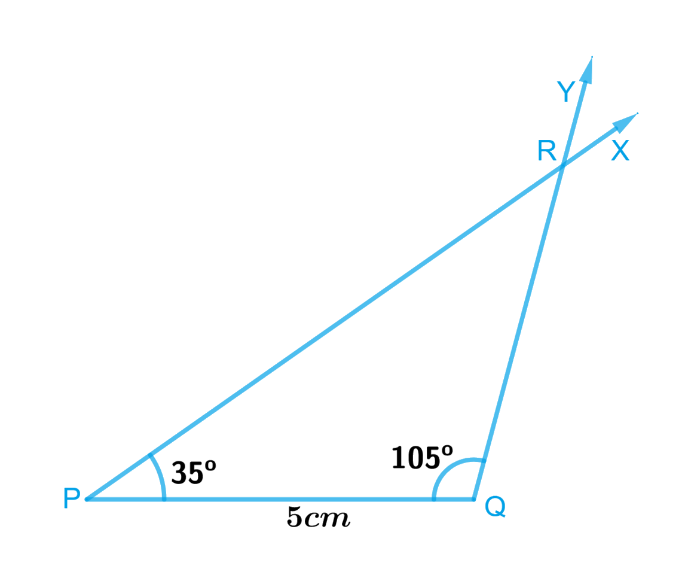# Ex.10.4 Q2 Practical Geometry Solution - NCERT Maths Class 7

Go back to  'Ex.10.4'

Construct $$ΔPQR$$  if  $$PQ = 5 \rm{cm}$$, $$m∠PQR = 105^\circ$$ and $$m∠QRP = 40^\circ$$. (Hint: Recall angle-sum property of a triangle).

What is known?

In triangle $$PQR$$ , $$PQ = 5 \rm{cm}$$, $$∠PQR = 105^\circ$$ and $$∠QRP = 40^\circ$$.

To construct:

A triangle $$ΔPQR$$ if $$PQ = 5 \rm{cm}$$, $$∠PQR = 105^\circ$$ and $$∠QRP = 40^\circ$$.

Reasoning:

We will use angle-sum property of a triangle to find the measure of $$∠RPQ$$
By angle sum property of a triangle,
$$∠PQR + ∠QRP + ∠RPQ = 180^\circ.$$
$$105^\circ + 40^\circ + ∠RPQ = 180^\circ.$$
So, $$∠RPQ = 35^\circ$$
Now, let’s Construct $$ΔPQR$$ such that $$PQ = 5\, {\rm{cm}} , ∠PQR = 105^\circ$$ and $$∠RPQ = 35^\circ$$, with the steps given below

Steps:Steps of construction

1. Draw a line segment $$PQ$$ of length $$5 \, \rm{cm}.$$
2. At $$P$$, draw $$PX$$ making $$35^\circ$$ with $$PQ.$$
3. At $$Q,$$ draw $$QY$$ making an angle of $$105^\circ$$ with $$PQ.$$
4. $$PX$$ and $$QY$$ will intersect at point $$R.$$

$$PQR$$ is the required triangle.